# DAC Essentials: The pursuit of perfection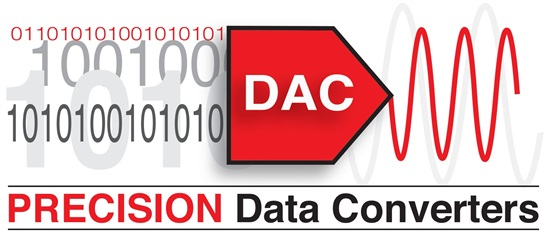In my last post, I introduced the DAC Essentials blog series here on Analog Wire. If you missed it, check it out here.

While most IC designers I know would shudder to think of a datasheet this way, the purpose of a datasheet is typically to explain how a device deviates from its ideal model. If it were possible for semiconductor suppliers to design and manufacture perfect, ideal operational amplifiers, for instance, we wouldn’t need op amp datasheets because everyone would know that they have very specific properties (infinite open-loop gain, infinite input impedance, etc). If only it were that simple.

Tony and I wanted to begin this blog series with a brief tutorial explaining the properties of an ideal DAC (digital-to-analog converter) before delving into its more complex specifications. The figure below illustrates the ideal DAC transfer function and highlights the parameters we’ll discuss.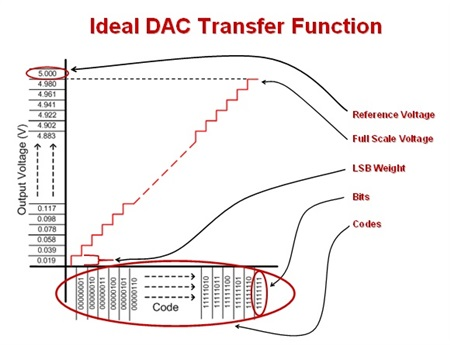The most fundamental property of any data converter, whether DAC or ADC (analog-to-digital converter), is its resolution. For a DAC, resolution describes the number of bits available in the digital domain to represent the analog output signal. With the resolution, we can calculate the number of codes, or total number of possible outputs, that we can write to the converter.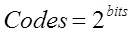The reference voltage applied to a data converter, whether internal or external, is very important. Any converter is only as good as its reference, as any noise or drift from the reference will be seen at the output. Note that we’ll address reference considerations in a future post, so stay tuned for more on that topic.

For a DAC, the reference voltage sets the output range and step size from code to code. Output step size from code to code is usually described as “least significant bit weight” or LSB weight. With the number of codes and reference voltage, we can calculate LSB weight as shown below. In an ideal DAC, every code-to-code transition is separated by exactly 1 LSB.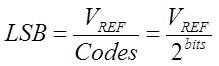It’s a little tricky to consider, but with n bits, the highest number we can actually count to is 2n – 1. If that leaves you scratching your head, consider the 2 bit case. With 2 bits, we can count 0, 1, 2, and 3 – but not 4 (24). This digital behavior is also consistent with the internal analog structure of most DACs, but we’ll get into that with a later post as well... have I convinced you to subscribe to the series yet? For now, take it on faith that the full-scale output range of a DAC can be calculated as shown below.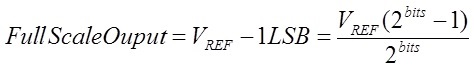Finally, we can define the ideal DAC transfer function as: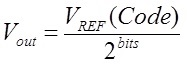Here are the key properties of the ideal DAC to remember:

• Near rail-to-rail output set by the reference input, remember the full scale output should be Vref - 1 LSB
• Any two sequential codes are exactly 1 LSB apart
• No missing codes, fully monotonic
• Instantaneous transition from code-to-code

In our next post, I’ll explain how real DACs deviate from the ideal DAC by discussing the static specifications used to describe linearity.

Leave your comments in the section below if you’d like to hear more about anything mentioned in this post, or if there is a topic you would like to see included in future posts!

Parents
• Aaron,

I am glad you have found the series helpful. Be sure to stick around for the remainder of the series and let us know if there is anything you would like to hear more about.

Comment
• Aaron,

I am glad you have found the series helpful. Be sure to stick around for the remainder of the series and let us know if there is anything you would like to hear more about.

Children
No Data